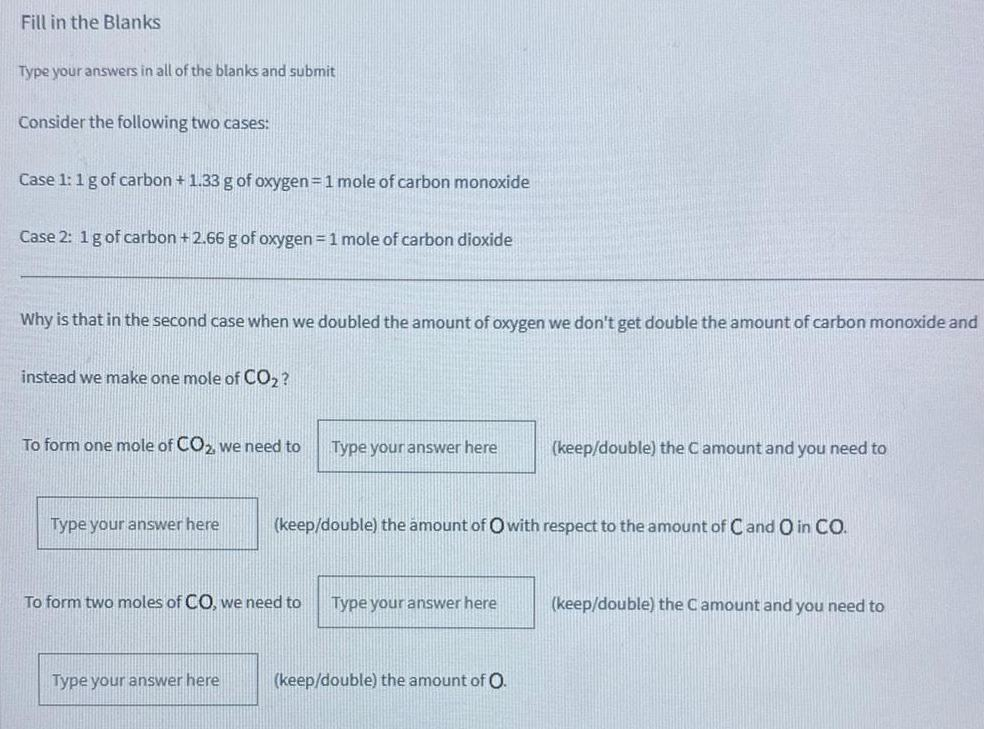Inorganic Chemistry
Metallurgy
Consider the following two cases: Case 1: 1 g of carbon + 1.33 g of oxygen = 1 mole of carbon monoxide Case 2: 1 g of carbon + 2.66 g of oxygen = 1 mole of carbon dioxide Why is that in the second case when we doubled the amount of oxygen we don't get double the amount of carbon monoxide and instead we make one mole of CO₂? To form one mole of CO2, we need to (keep/double) the C amount and you need to (keep/double) the amount of Owith respect to the amount of C and O in CO. To form two moles of CO, we need to (keep/double) the C amount and you need to (keep/double) the amount of O.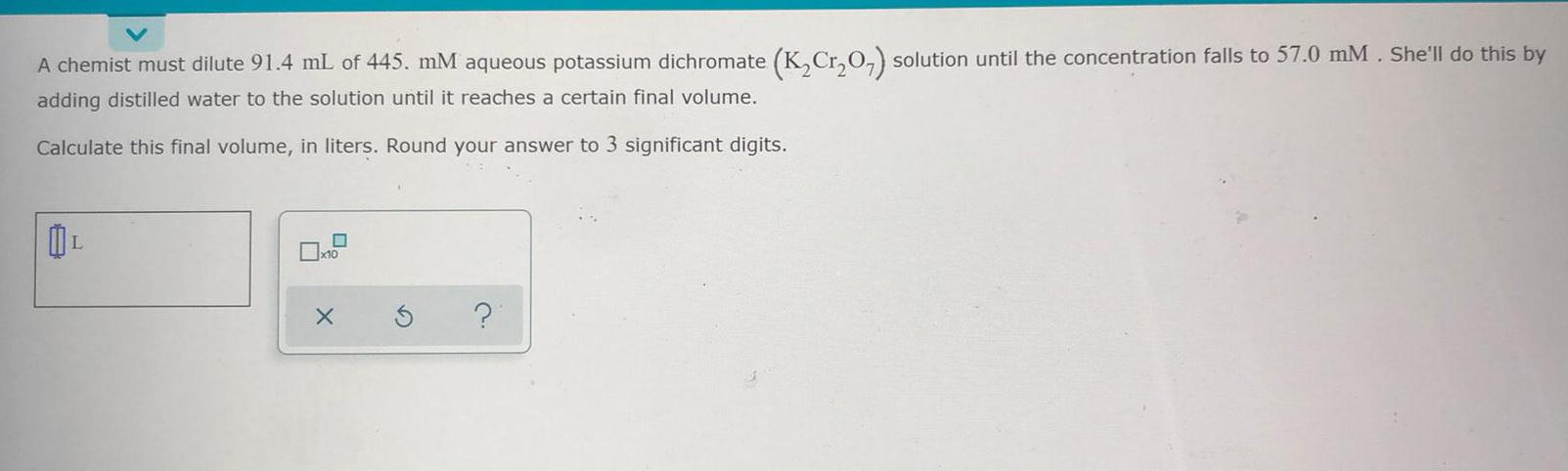Inorganic Chemistry
Metallurgy
A chemist must dilute 91.4 mL of 445. mM aqueous potassium dichromate (K₂Cr₂O7) solution until the concentration falls to 57.0 mM . She'll do this by adding distilled water to the solution until it reaches a certain final volume. Calculate this final volume, in liters. Round your answer to 3 significant digits.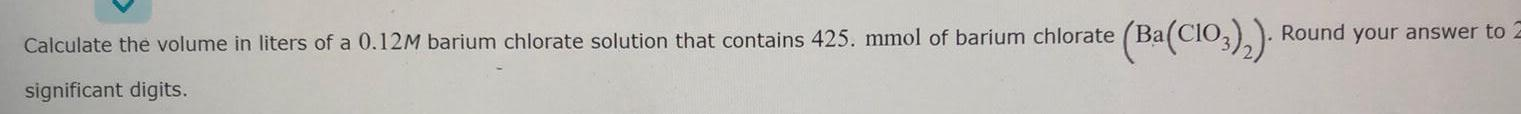Inorganic Chemistry
Metallurgy
Calculate the volume in liters of a 0.12M barium chlorate solution that contains 425. mmol of barium chlorate (Ba(CIO3)₂). Round your answer to 2 significant digits.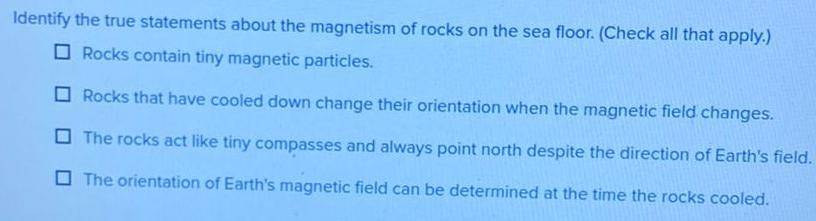Inorganic Chemistry
Metallurgy
Identify the true statements about the magnetism of rocks on the sea floor. (Check all that apply.) Rocks contain tiny magnetic particles. Rocks that have cooled down change their orientation when the magnetic field changes. The rocks act like tiny compasses and always point north despite the direction of Earth's field. The orientation of Earth's magnetic field can be determined at the time the rocks cooled.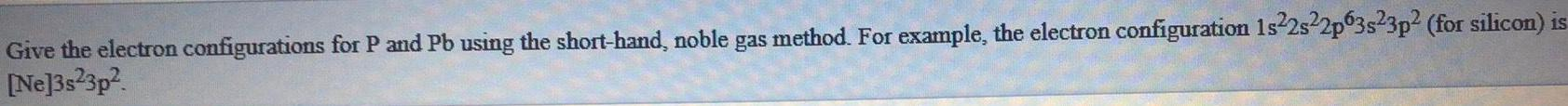Inorganic Chemistry
Metallurgy
Give the electron configurations for P and Pb using the short-hand, noble gas method. For example, the electron configuration 1s22s22p63s23p2 (for silicon) is [Ne]3s²3p²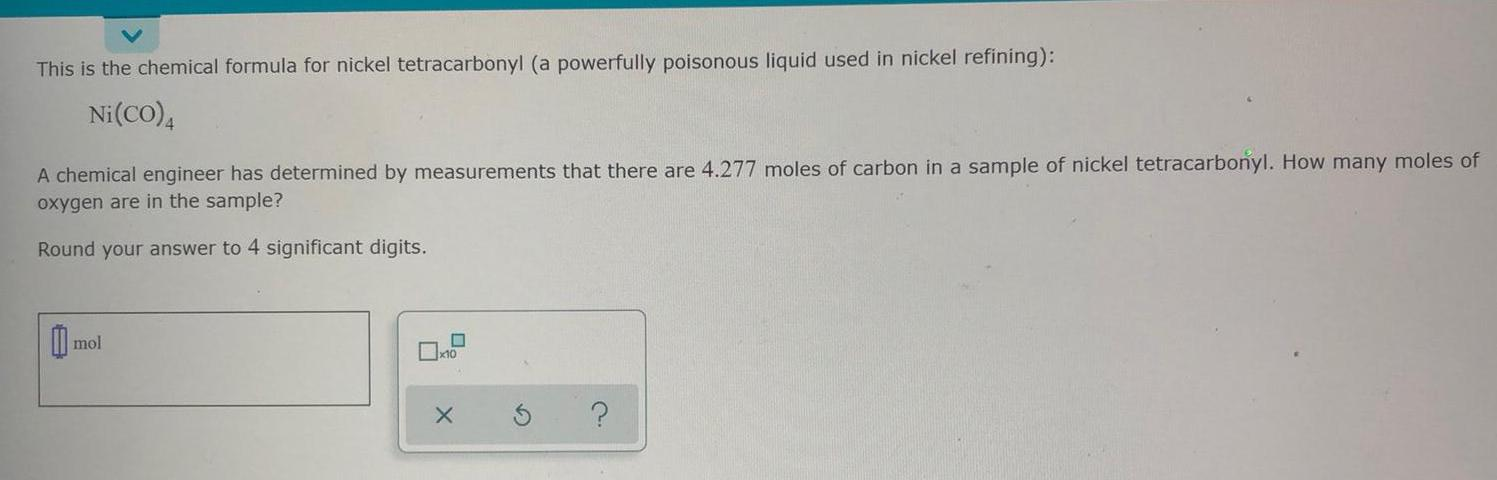Inorganic Chemistry
Metallurgy
This is the chemical formula for nickel tetracarbonyl (a powerfully poisonous liquid used in nickel refining): Ni(CO)4 A chemical engineer has determined by measurements that there are 4.277 moles of carbon in a sample of nickel tetracarbonyl. How many moles of oxygen are in the sample? Round your answer to 4 significant digits.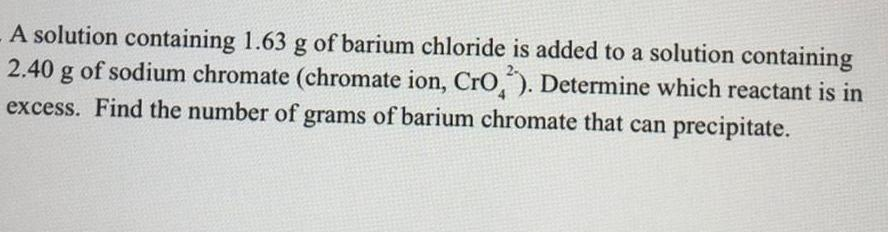Inorganic Chemistry
Metallurgy
A solution containing 1.63 g of barium chloride is added to a solution containing 2.40 g of sodium chromate (chromate ion, CrO2). Determine which reactant is in excess. Find the number of grams of barium chromate that can precipitate.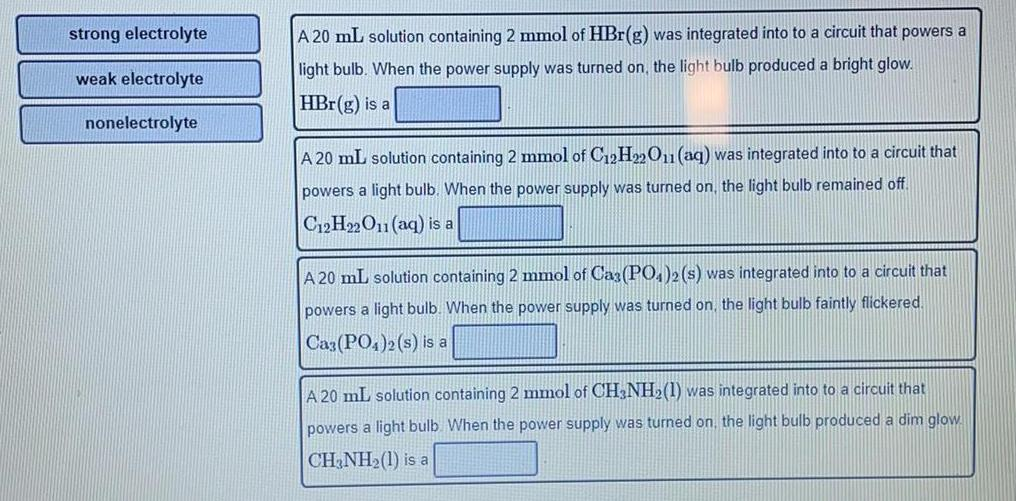Inorganic Chemistry
Metallurgy
strong electrolyte weak electrolyte nonelectrolyte A 20 mL solution containing 2 mmol of HBr(g) was integrated into to a circuit that powers a light bulb. When the power supply was turned on, the light bulb produced a bright glow. HBr (g) is a A 20 mL solution containing 2 mmol of C12H22O11 (aq) was integrated into to a circuit that powers a light bulb. When the power supply was turned on, the light bulb remained off. C12H22O11 (aq) is a A 20 mL solution containing 2 mmol of Ca3(PO4)2 (s) was integrated into to a circuit that powers a light bulb. When the power supply was turned on, the light bulb faintly flickered. Ca3(PO4)2 (s) is a A 20 mL solution containing 2 mmol of CH3NH₂(1) was integrated into to a circuit that powers a light bulb. When the power supply was turned on, the light bulb produced a dim glow. CH3NH₂(l) is aInorganic Chemistry
Metallurgy
In two or more complete sentences, describe how one of the occupations that you studied would use descriptive statistics when performing their job. Justify your answers in two or more complete sentences.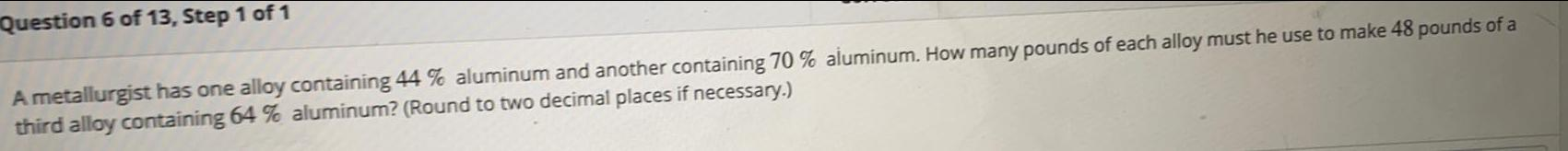Inorganic Chemistry
Metallurgy
A metallurgist has one alloy containing 44 % aluminum and another containing 70 % aluminum. How many pounds of each alloy must he use to make 48 pounds of a third alloy containing 64 % aluminum? (Round to two decimal places if necessary.)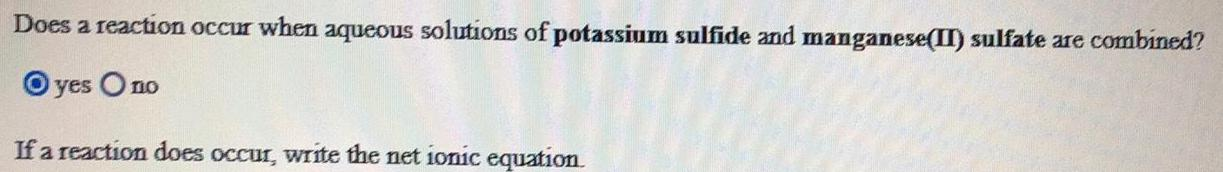Inorganic Chemistry
Metallurgy
Does a reaction occur when aqueous solutions of potassium sulfide and manganese(II) sulfate are combined? yes / no If a reaction does occur, write the net ionic equation.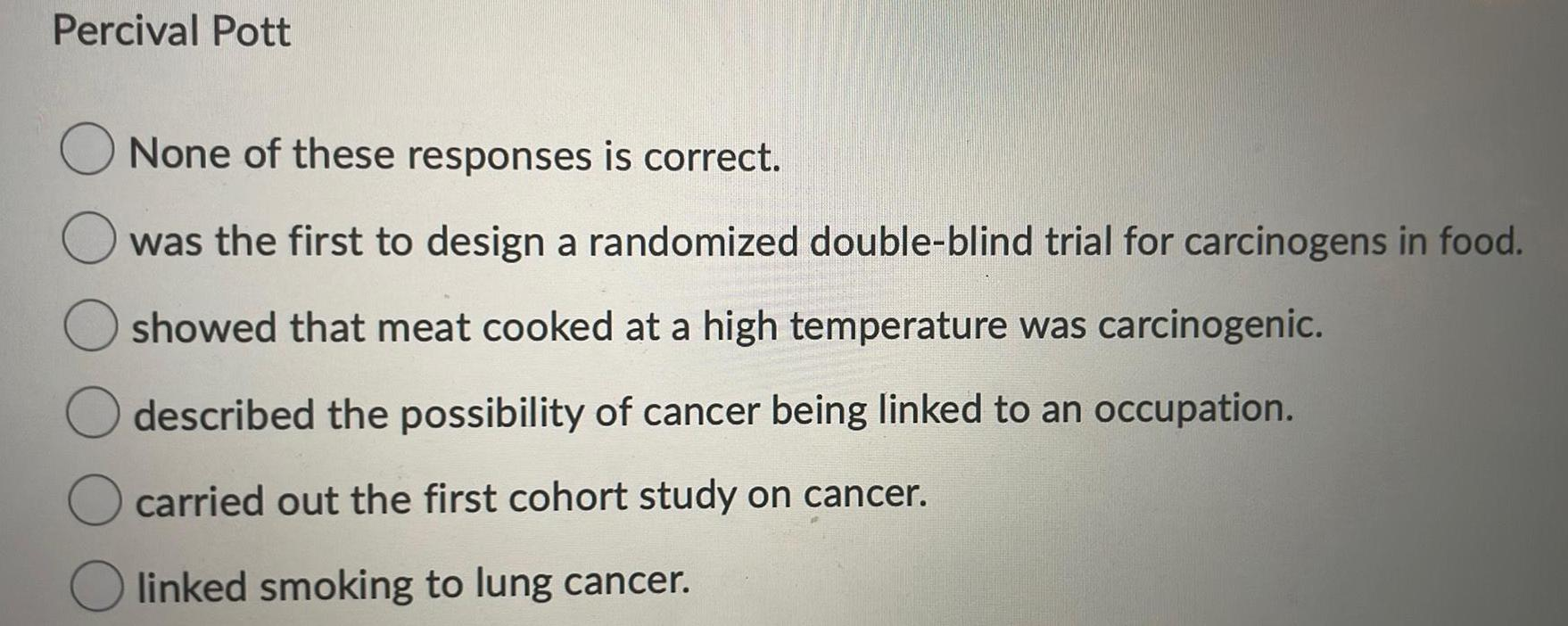Inorganic Chemistry
Metallurgy
Percival Pott None of these responses is correct. was the first to design a randomized double-blind trial for carcinogens in food. showed that meat cooked at a high temperature was carcinogenic. described the possibility of cancer being linked to an occupation. carried out the first cohort study on cancer. linked smoking to lung cancer.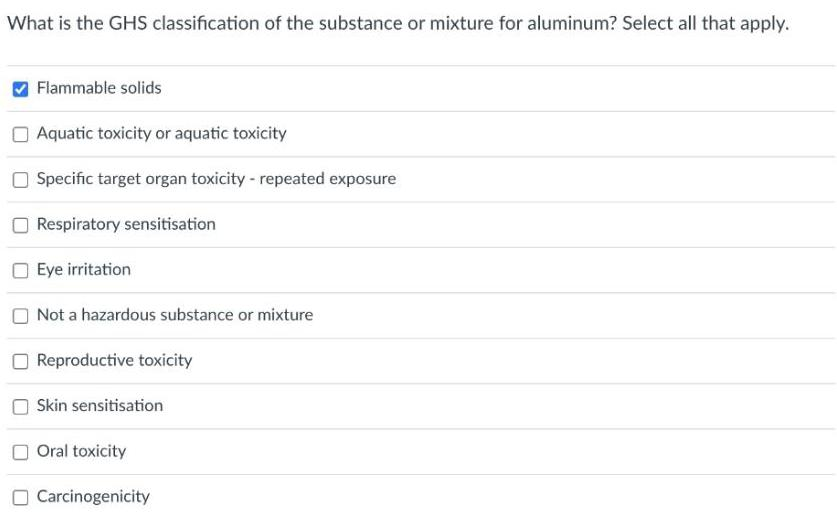Inorganic Chemistry
Metallurgy
What is the GHS classification of the substance or mixture for aluminum? Select all that apply. Flammable solids Aquatic toxicity or aquatic toxicity Specific target organ toxicity - repeated exposure Respiratory sensitisation Eye irritation Not a hazardous substance or mixture Reproductive toxicity Skin sensitisation Oral toxicity Carcinogenicity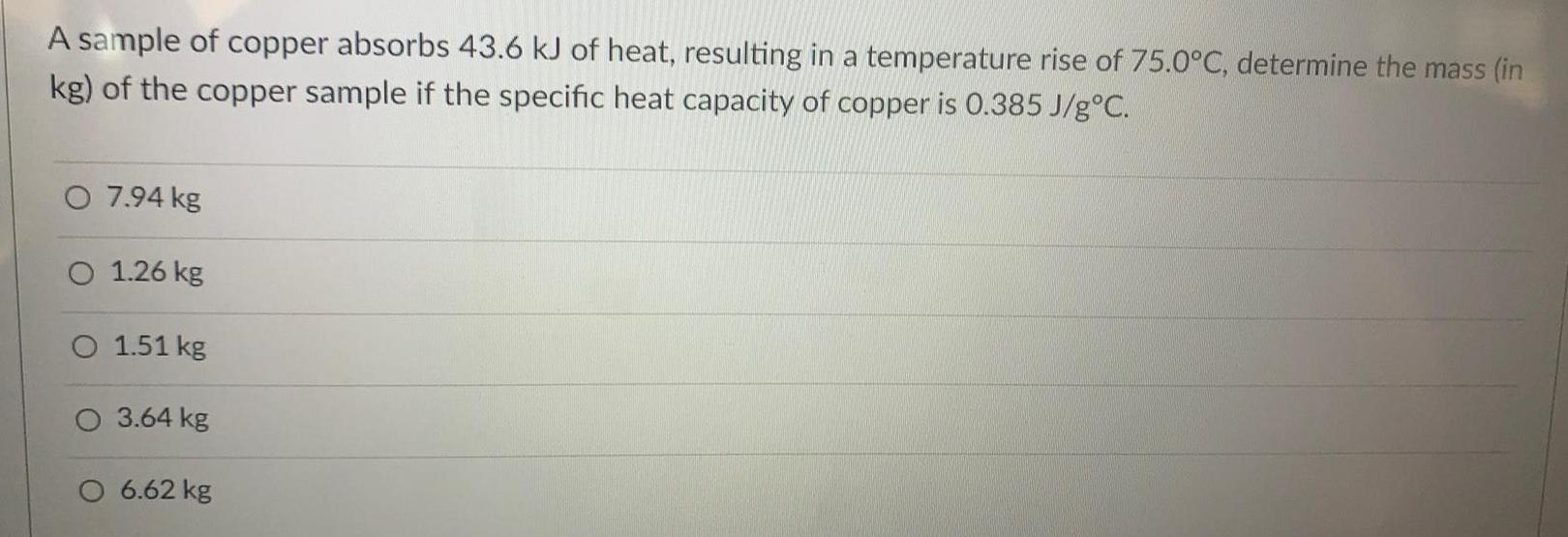Inorganic Chemistry
Metallurgy
A sample of copper absorbs 43.6 kJ of heat, resulting in a temperature rise of 75.0°C, determine the mass (in kg) of the copper sample if the specific heat capacity of copper is 0.385 J/g°C. 7.94 kg 1.26 kg 1.51 kg 3.64 kg 6.62 kg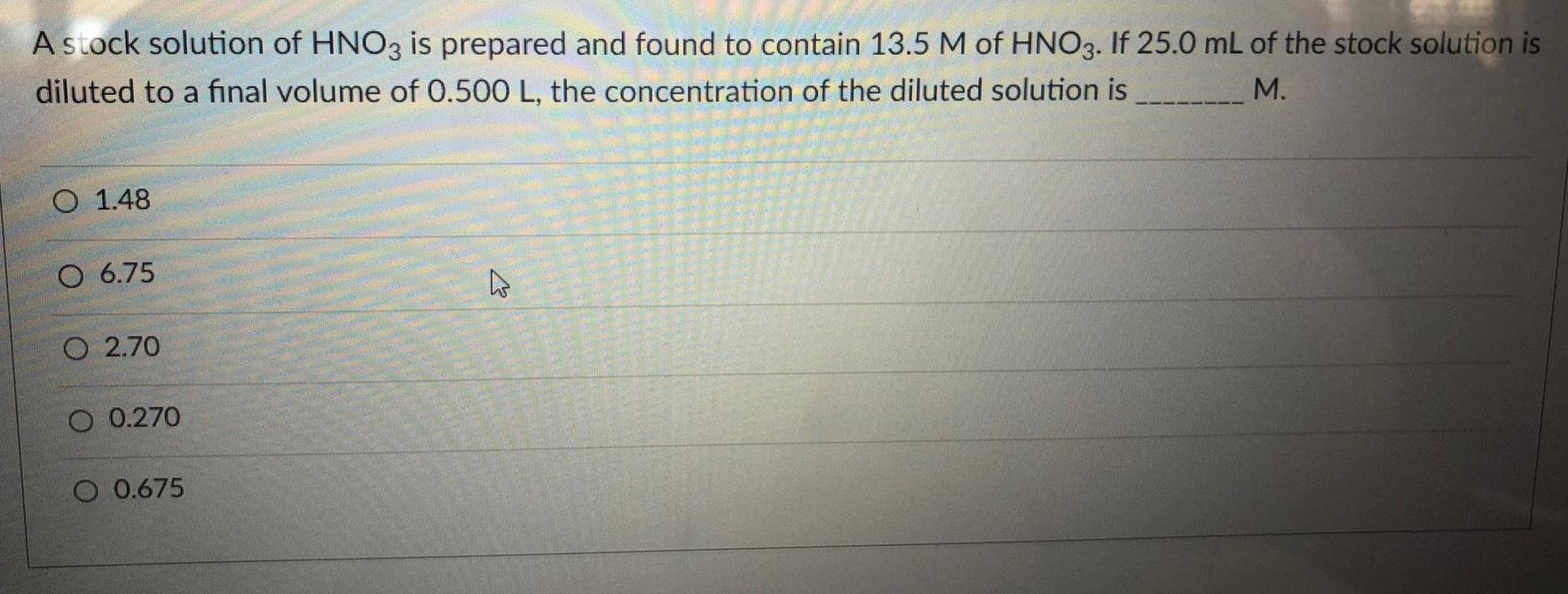Inorganic Chemistry
Metallurgy
A stock solution of HNO3 is prepared and found to contain 13.5 M of HNO3. If 25.0 mL of the stock solution is diluted to a final volume of 0.500 L, the concentration of the diluted solution is ---M. 1.48 6.75 2.70 0.270 0.675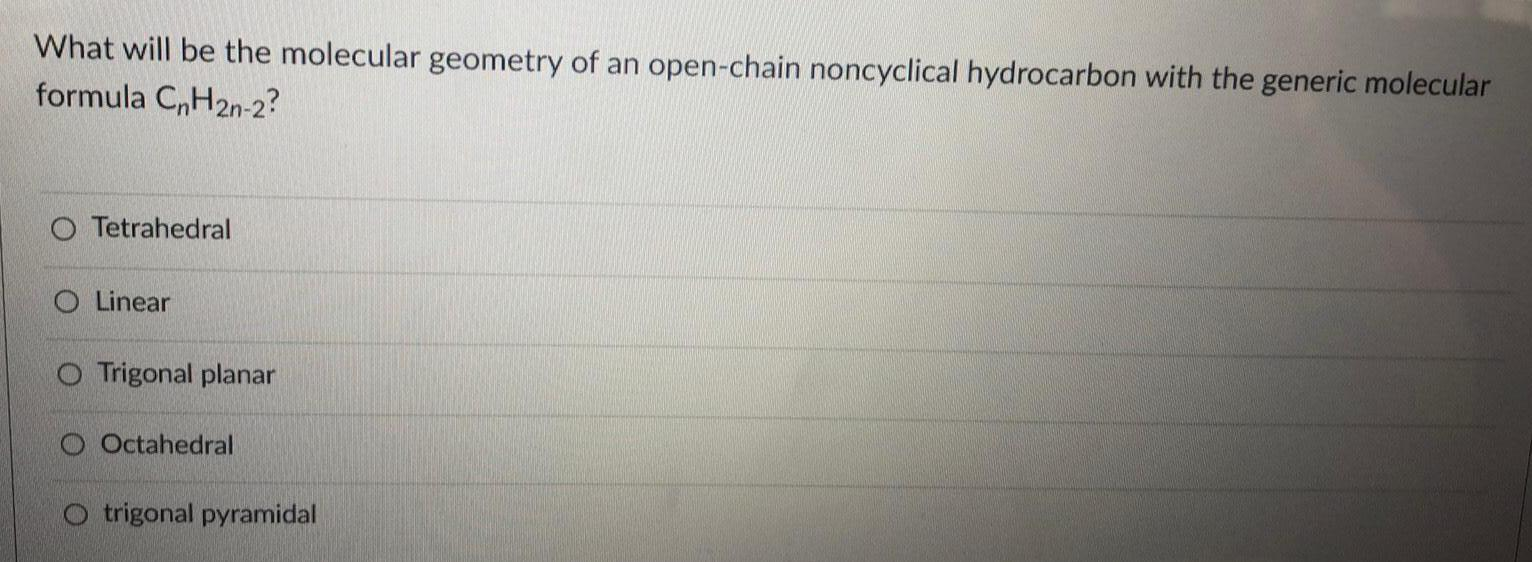Inorganic Chemistry
Metallurgy
What will be the molecular geometry of an open-chain noncyclical hydrocarbon with the generic molecular formula CnH2n-2? Tetrahedral Linear Trigonal planar Octahedral trigonal pyramidal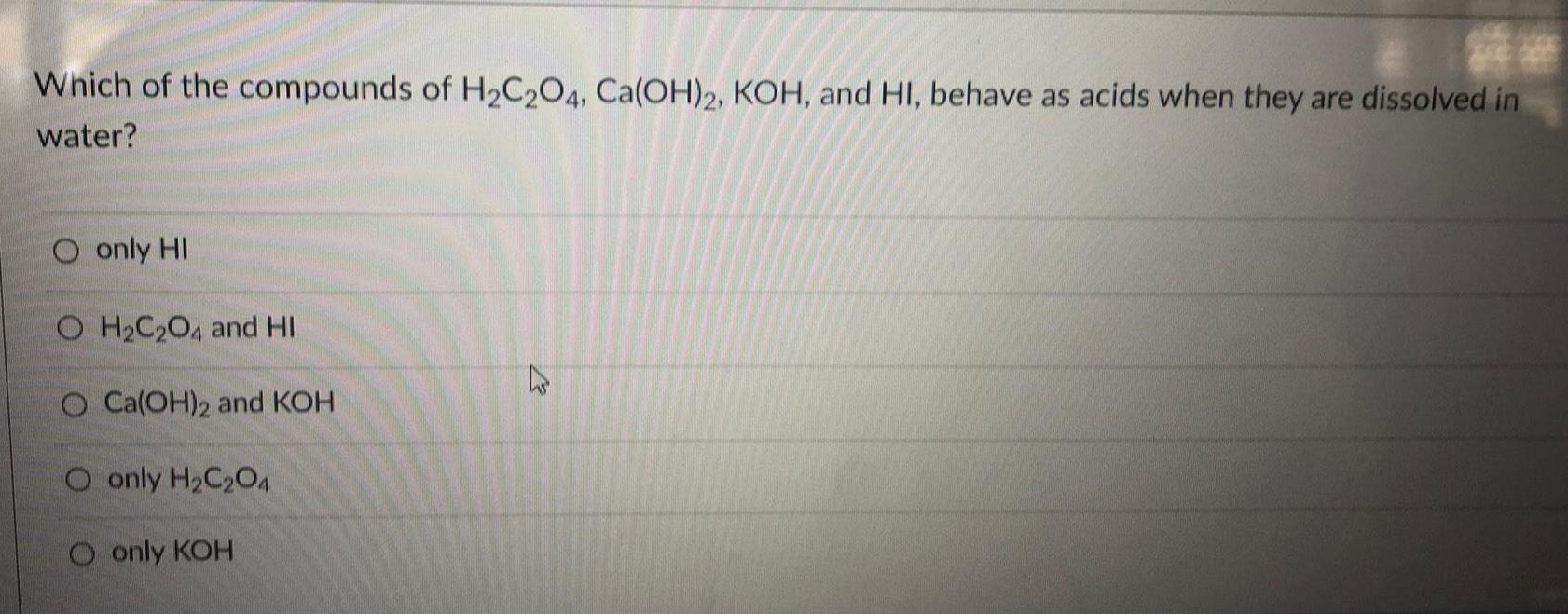Inorganic Chemistry
Metallurgy
Which of the compounds of H₂C2O4, Ca(OH)2, KOH, and HI, behave as acids when they are dissolved in water? only HI H₂C2O4 and HI Ca(OH)2 and KOH only H₂C2O4 only KOH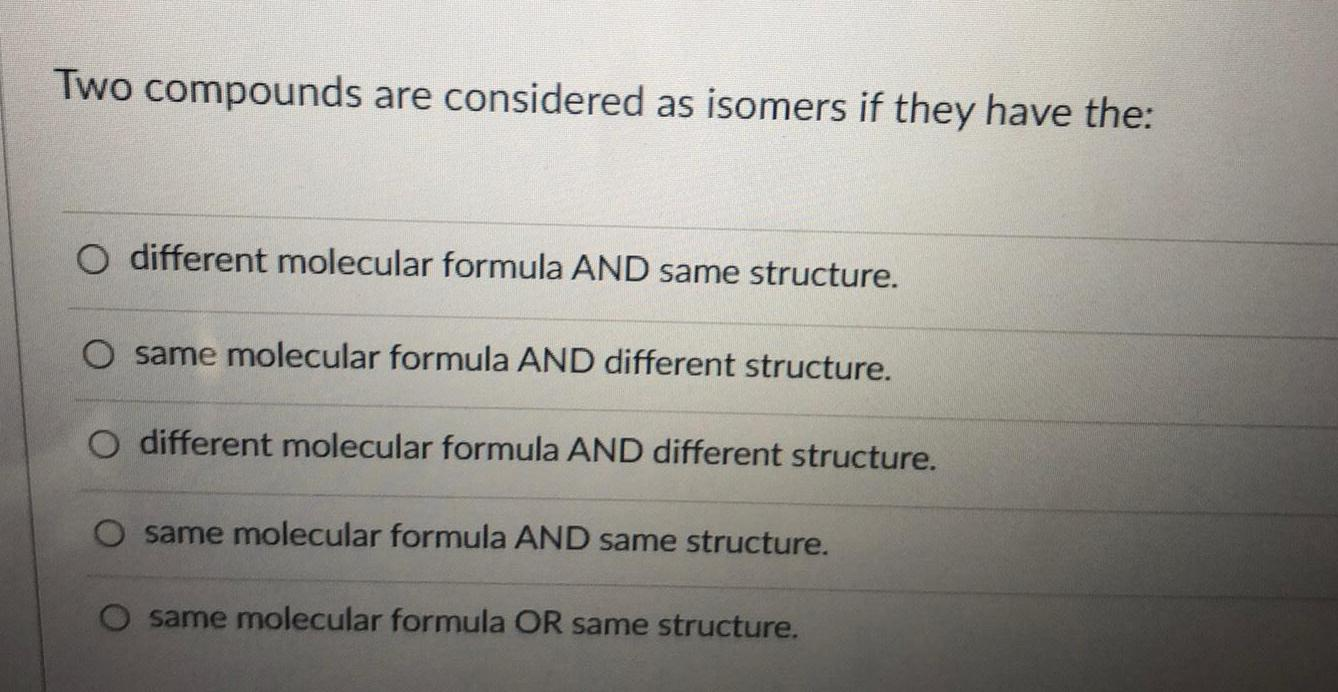Inorganic Chemistry
Metallurgy
Two compounds are considered as isomers if they have the: different molecular formula AND same structure. same molecular formula AND different structure. different molecular formula AND different structure. same molecular formula AND same structure. same molecular formula OR same structure.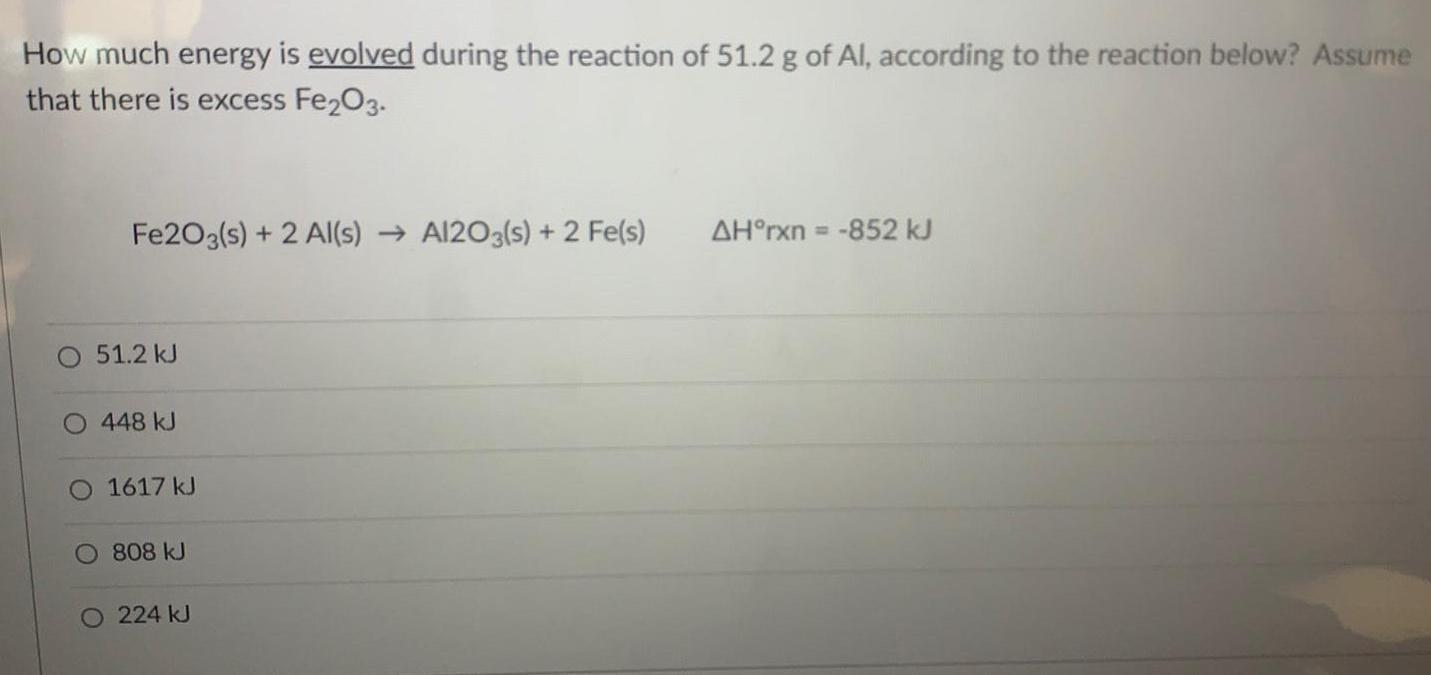Inorganic Chemistry
Metallurgy
How much energy is evolved during the reaction of 51.2 g of Al, according to the reaction below? Assume that there is excess Fe₂O3. Fe2O3(s) + 2 Al(s) → Al2O3(s) + 2 Fe(s) ΔH°rxn= -852 kJ 51.2 kJ 448 kJ 1617 kJ 808 kJ 224 kJ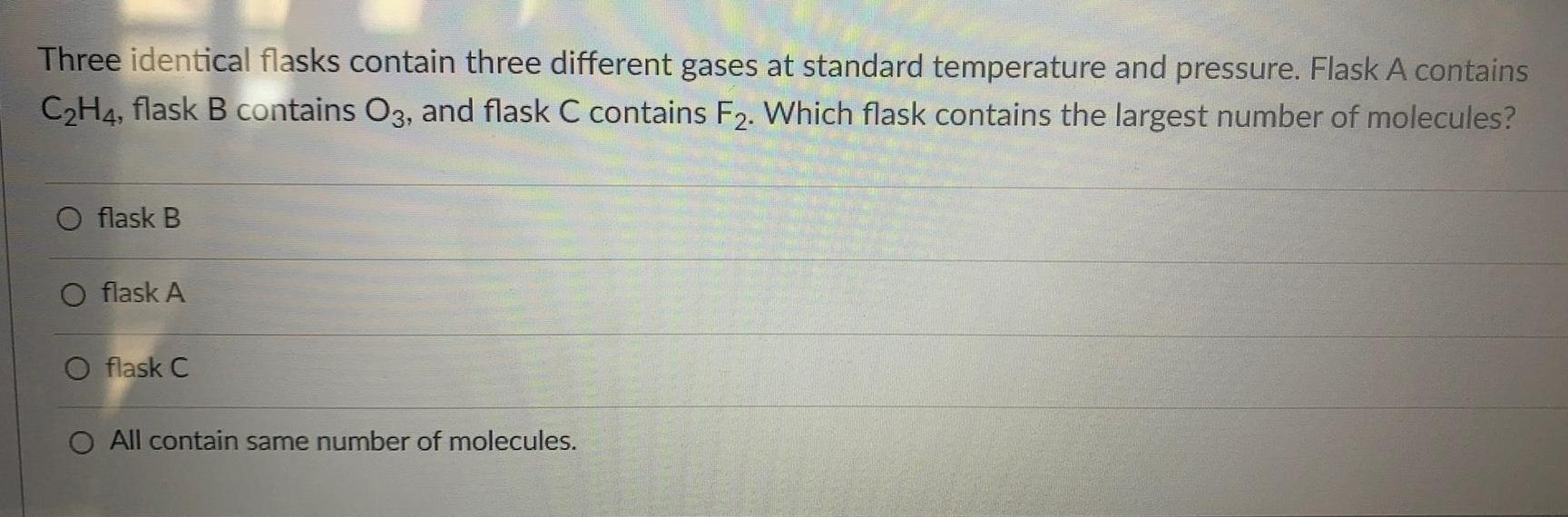Inorganic Chemistry
Metallurgy
Three identical flasks contain three different gases at standard temperature and pressure. Flask A contains C₂H4, flask B contains O3, and flask C contains F2. Which flask contains the largest number of molecules? flask B flask A flask C All contain same number of molecules.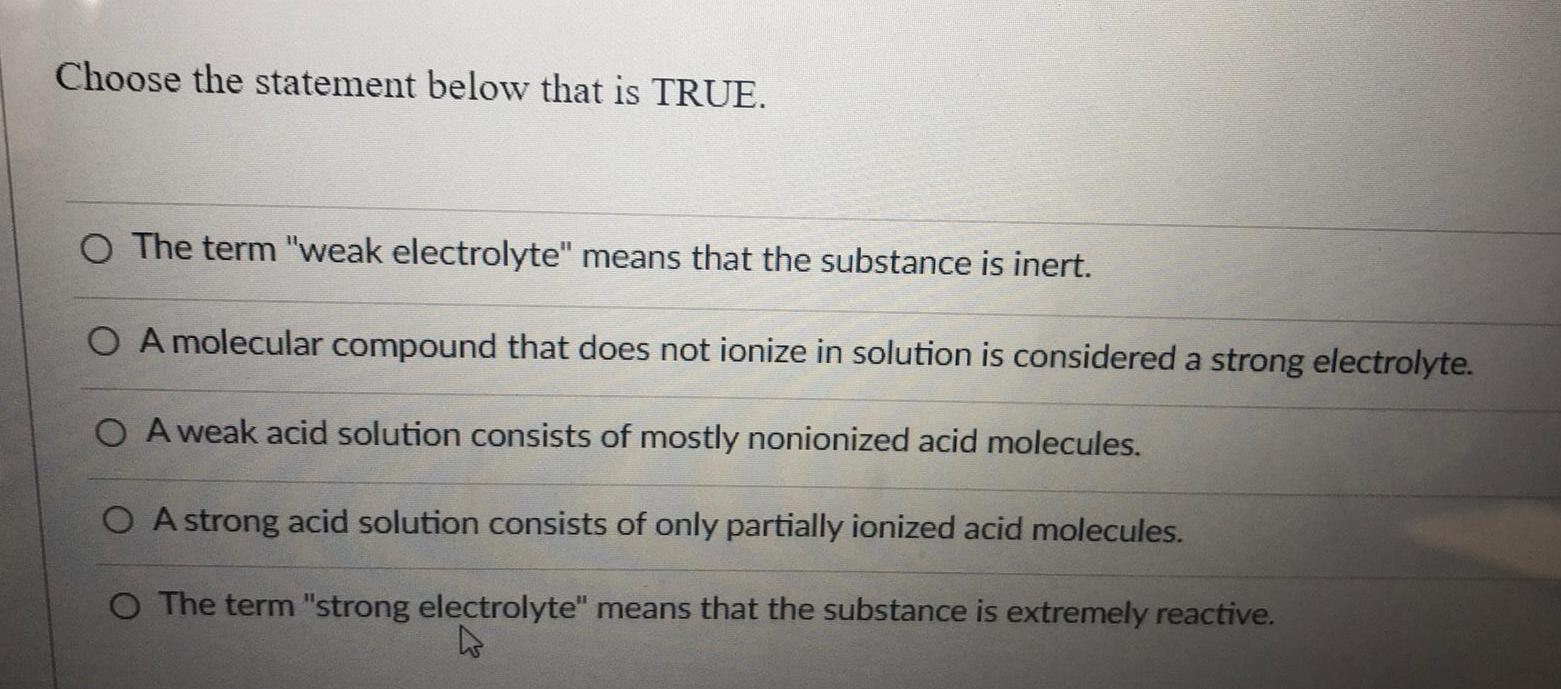Inorganic Chemistry
Metallurgy
Choose the statement below that is TRUE. The term "weak electrolyte" means that the substance is inert. A molecular compound that does not ionize in solution is considered a strong electrolyte. A weak acid solution consists of mostly nonionized acid molecules. A strong acid solution consists of only partially ionized acid molecules. The term "strong electrolyte" means that the substance is extremely reactive.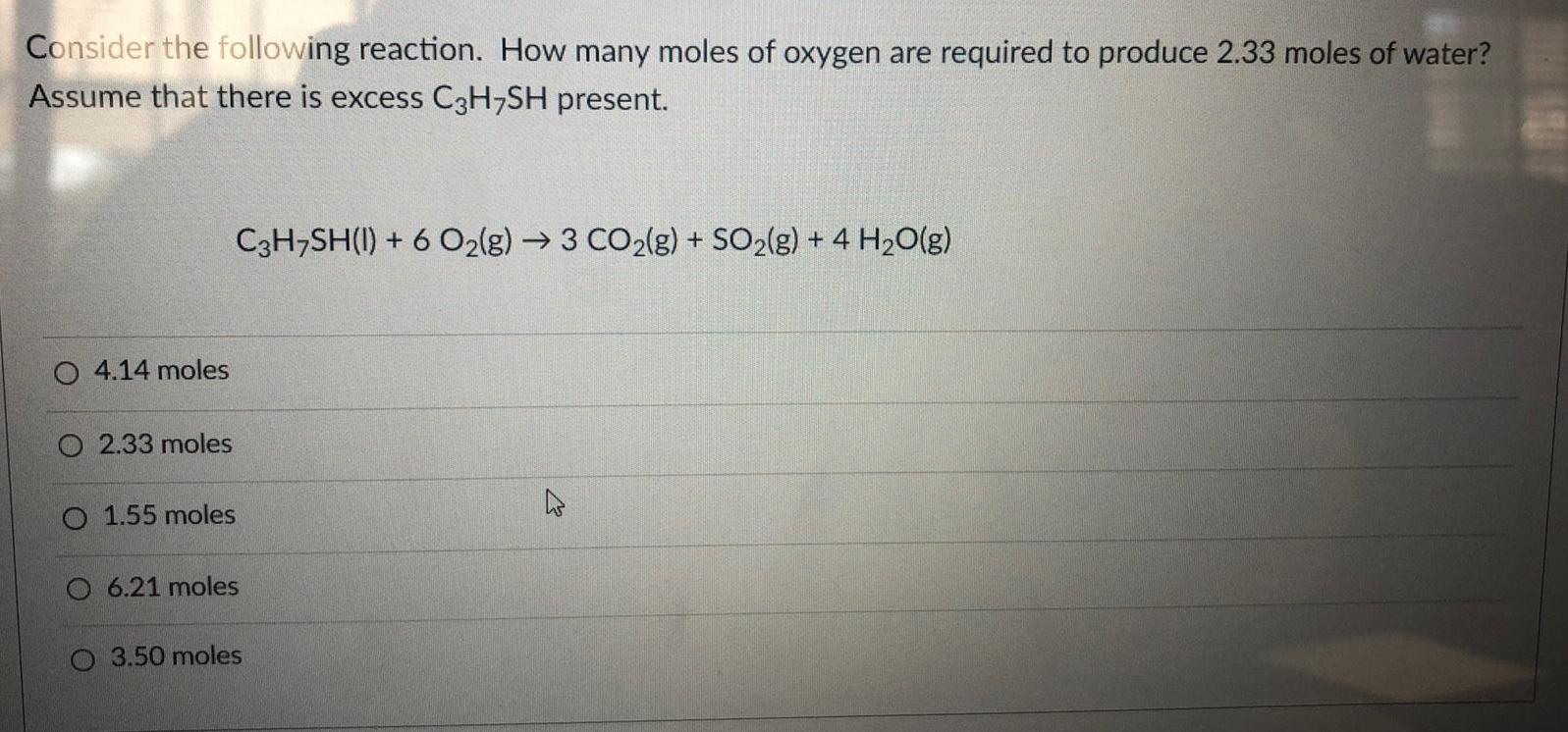Inorganic Chemistry
Metallurgy
Consider the following reaction. How many moles of oxygen are required to produce 2.33 moles of water? Assume that there is excess C3H7SH present. C3H7SH(l) + 6 O₂(g) → 3 CO2(g) + SO2(g) + 4H₂O(g) 4.14 moles 2.33 moles 1.55 moles 6.21 moles 3.50 moles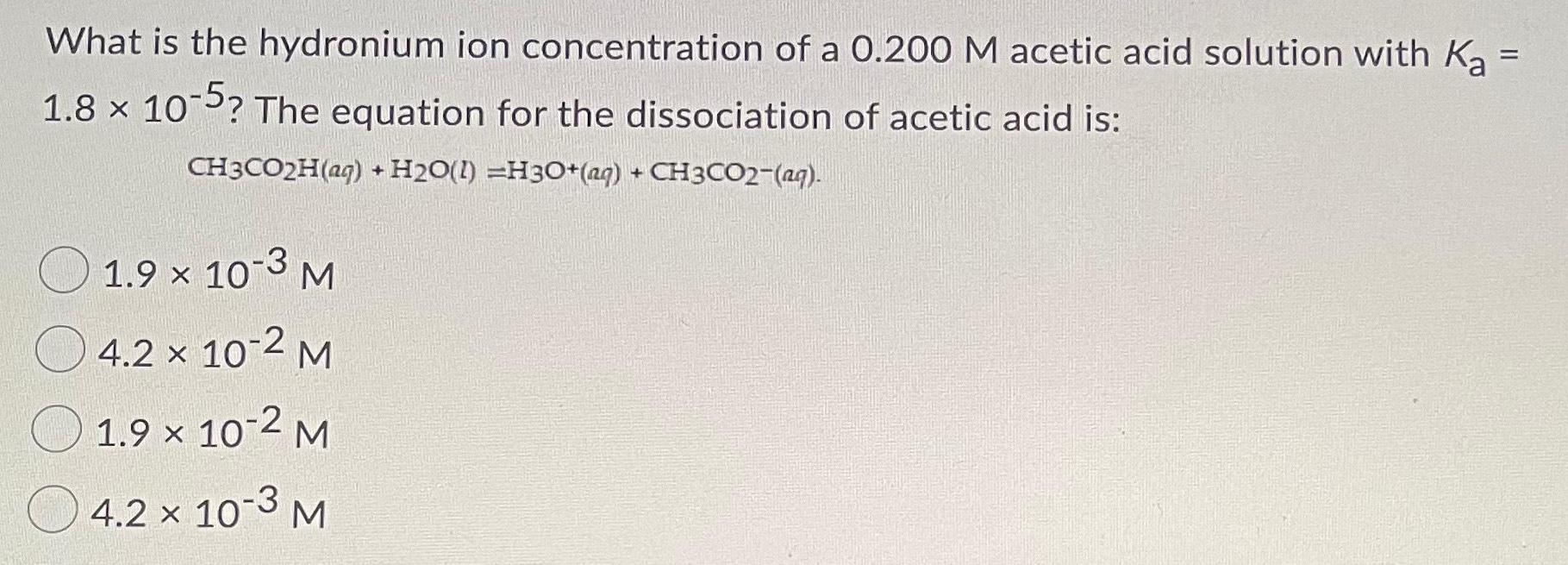Inorganic Chemistry
Metallurgy
What is the hydronium ion concentration of a 0.200 M acetic acid solution with Ka = 1.8 x 10-5? The equation for the dissociation of acetic acid is: CH3CO2H(aq) + H₂O(I) =H3O+ (aq) + CH3CO2−(aq). 1.9 × 10-3 M 4.2 x 10-2 M 1.9 × 10-2 M 4.2 × 10-3 M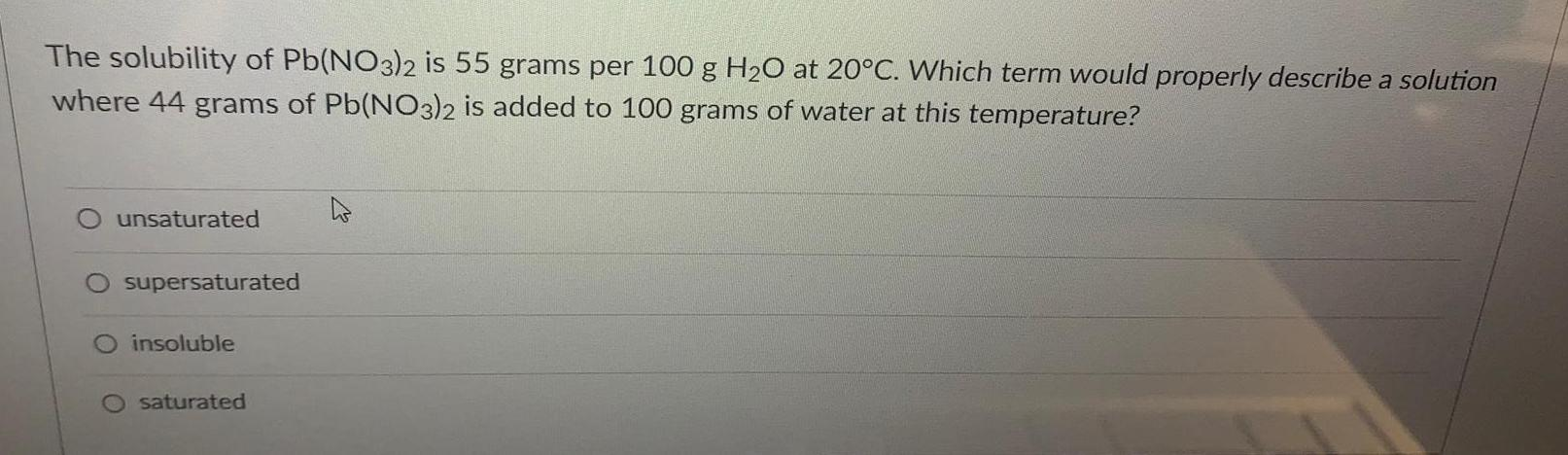Inorganic Chemistry
Metallurgy
The solubility of Pb(NO3)2 is 55 grams per 100 g H₂O at 20°C. Which term would properly describe a solution where 44 grams of Pb(NO3)2 is added to 100 grams of water at this temperature? unsaturated supersaturated insoluble saturated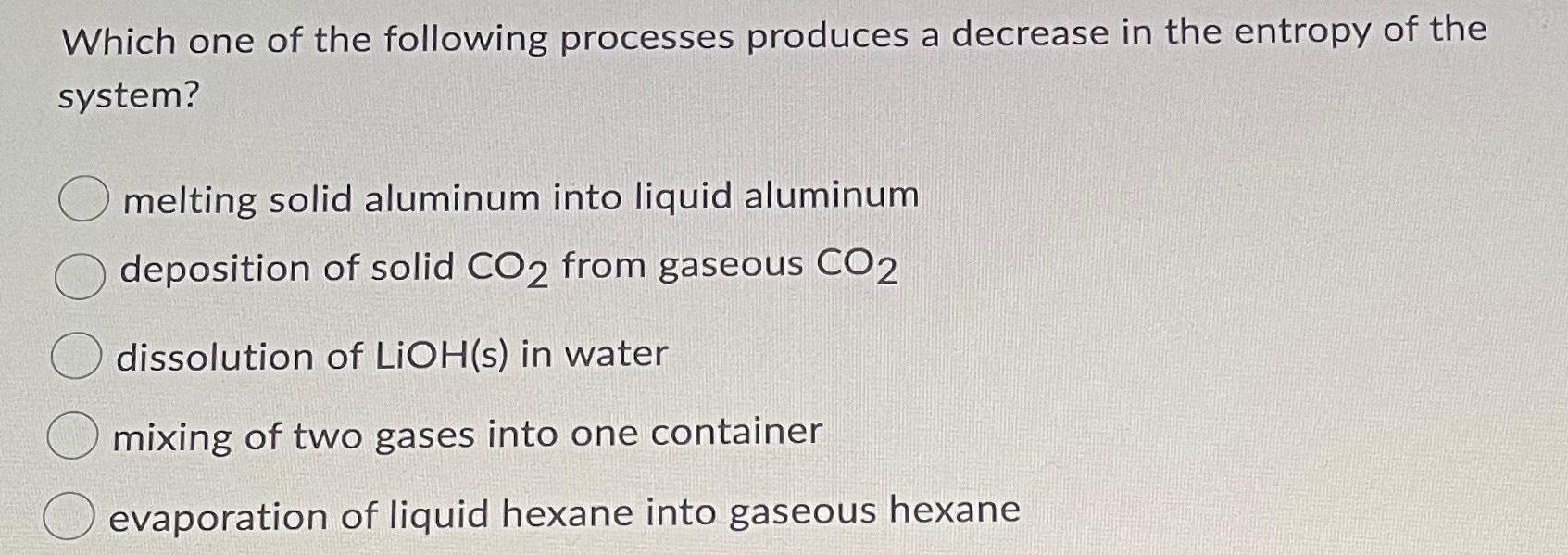Inorganic Chemistry
Metallurgy
Which one of the following processes produces a decrease in the entropy of the system? melting solid aluminum into liquid aluminum deposition of solid CO2 from gaseous CO2 dissolution of LiOH(s) in water mixing of two gases into one container evaporation of liquid hexane into gaseous hexane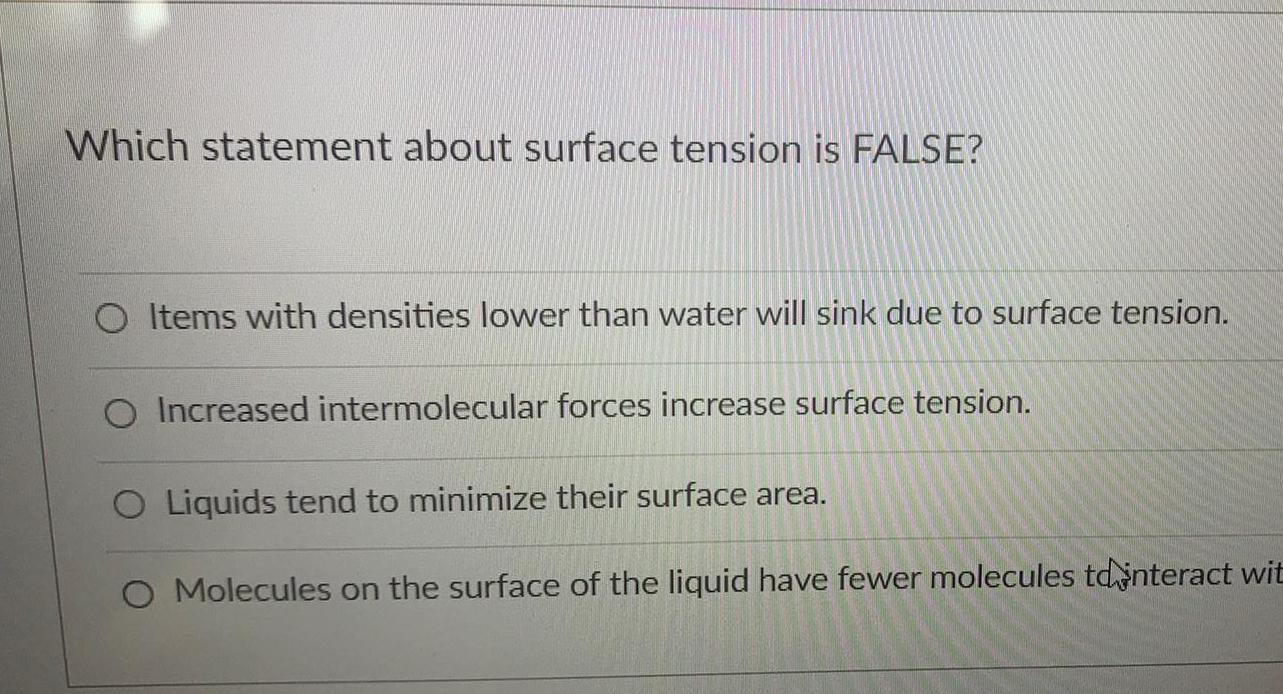Inorganic Chemistry
Metallurgy
Which statement about surface tension is FALSE? Items with densities lower than water will sink due to surface tension. Increased intermolecular forces increase surface tension. Liquids tend to minimize their surface area. Molecules on the surface of the liquid have fewer molecules to interact wit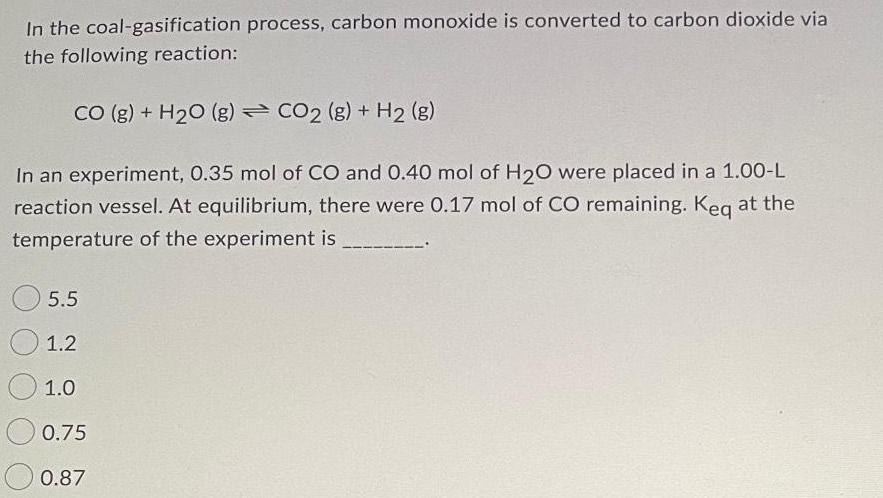Inorganic Chemistry
Metallurgy
In the coal-gasification process, carbon monoxide is converted to carbon dioxide via the following reaction: CO (g) + H2O (g) → CO2 (g) + H2 (g) In an experiment, 0.35 mol of CO and 0.40 mol of H₂O were placed in a 1.00-L reaction vessel. At equilibrium, there were 0.17 mol of CO remaining. Keq at the temperature of the experiment is_____________. 5.5 1.2 1.0 0.75 0.87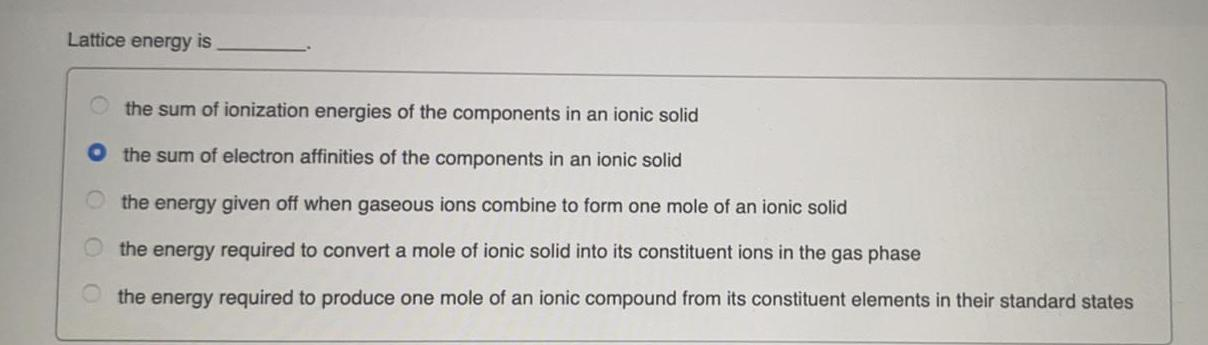Inorganic Chemistry
Metallurgy
Lattice energy is _____ the sum of ionization energies of the components in an ionic solid the sum of electron affinities of the components in an ionic solid the energy given off when gaseous ions combine to form one mole of an ionic solid the energy required to convert a mole of ionic solid into its constituent ions in the gas phase the energy required to produce one mole of an ionic compound from its constituent elements in their standard states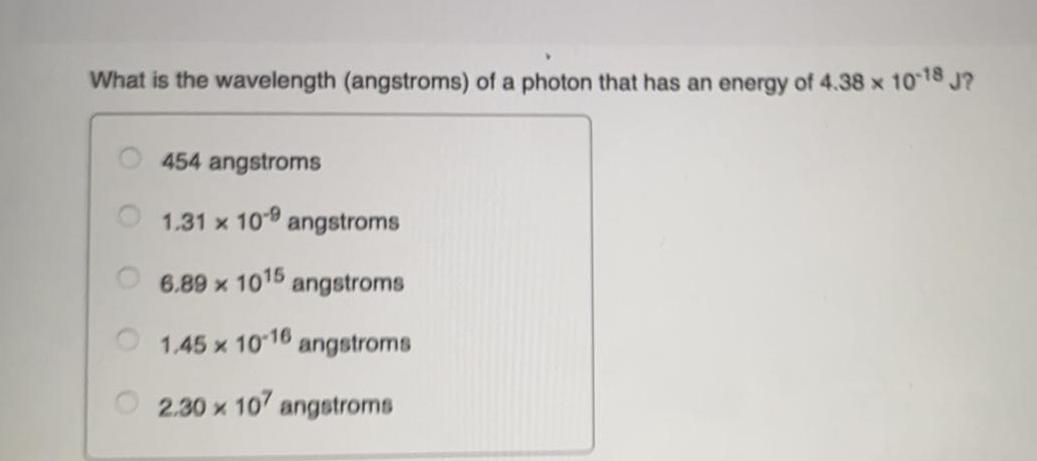Inorganic Chemistry
Metallurgy
What is the wavelength (angstroms) of a photon that has an energy of 4.38 x 10^-18 J? 454 angstroms 1.31 x 10^9 angstroms 6.89 x 10^15 angstroms 1.45 x 10^-16 angstroms 2.30 x 10^7 angstroms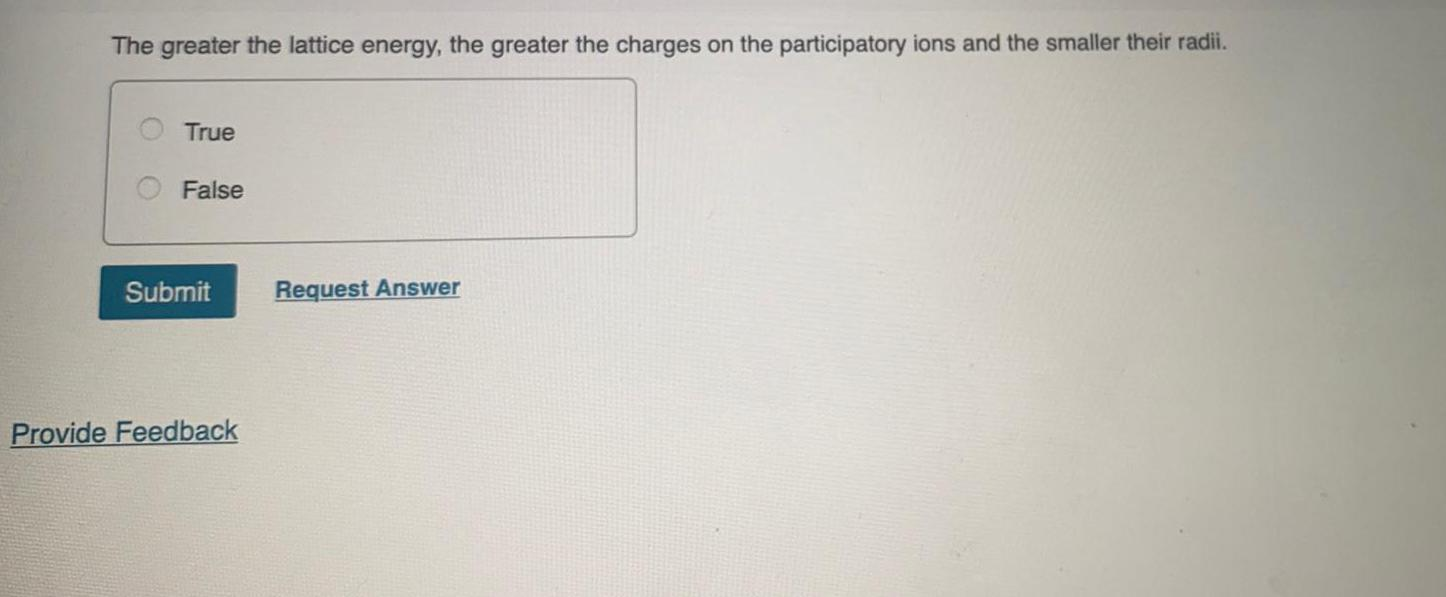Inorganic Chemistry
Metallurgy
The greater the lattice energy, the greater the charges on the participatory ions and the smaller their radii. True False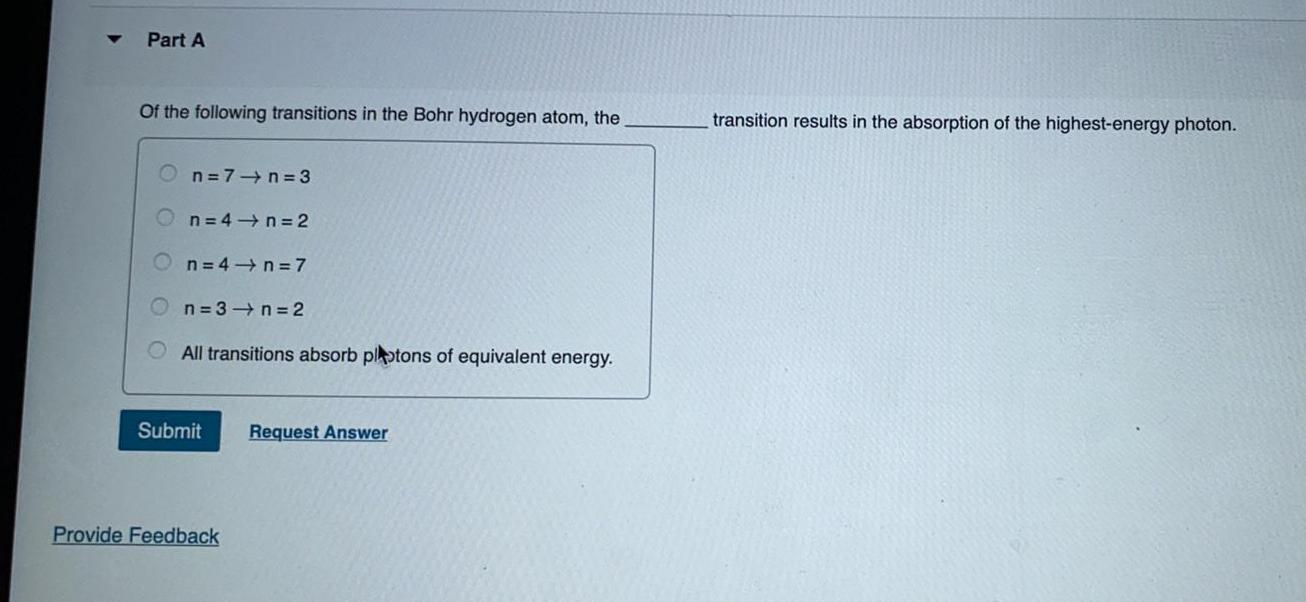Inorganic Chemistry
Metallurgy
Of the following transitions in the Bohr hydrogen atom, the n=7→n=3 n=4 →n=2 n=4 → n = 7 n=3→n=2 All transitions absorb plotons of equivalent energy.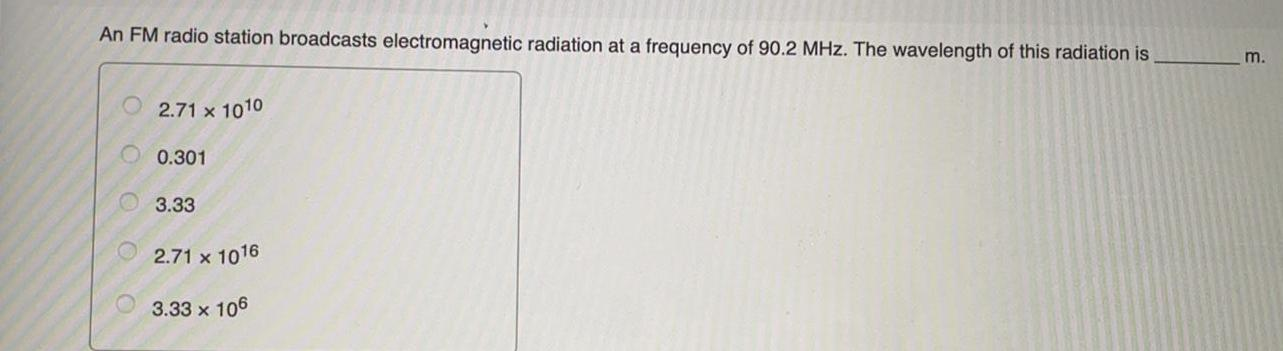Inorganic Chemistry
Metallurgy
An FM radio station broadcasts electromagnetic radiation at a frequency of 90.2 MHz. The wavelength of this radiation is 2.71 x 10^10 0.301 3.33 2.71 x 10^16 3.33 x 10^6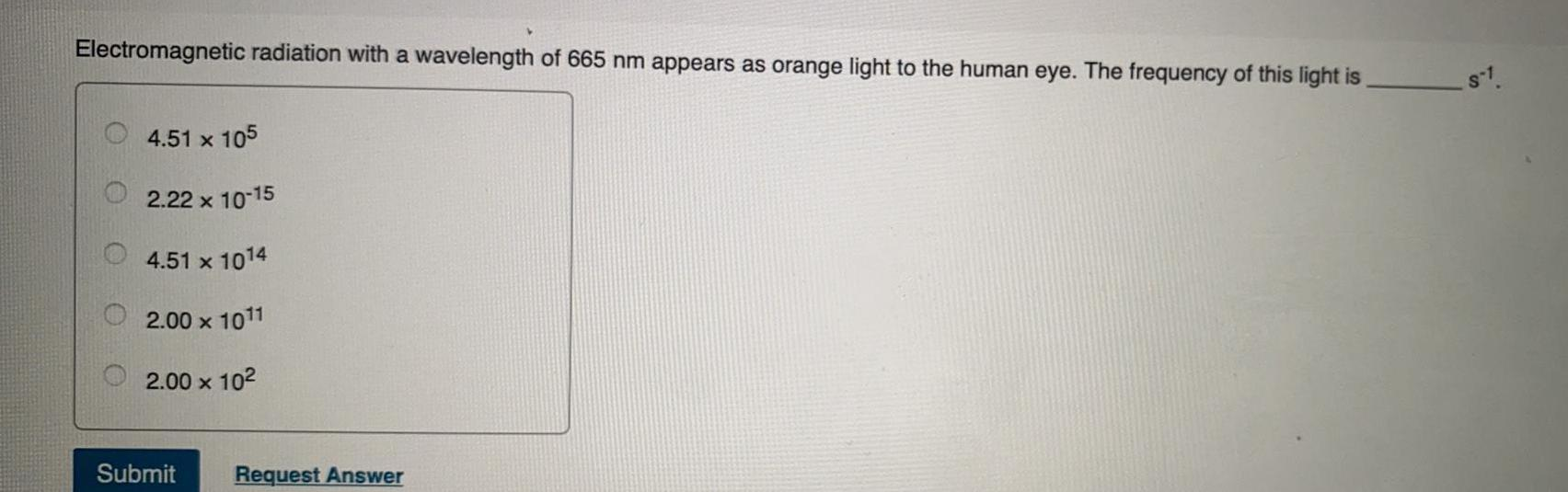Inorganic Chemistry
Metallurgy
Electromagnetic radiation with a wavelength of 665 nm appears as orange light to the human eye. The frequency of this light is 4.51 x 10^5 2.22 x 10^-15 4.51 x 10^14 2.00 x 10^11 2.00 × 10²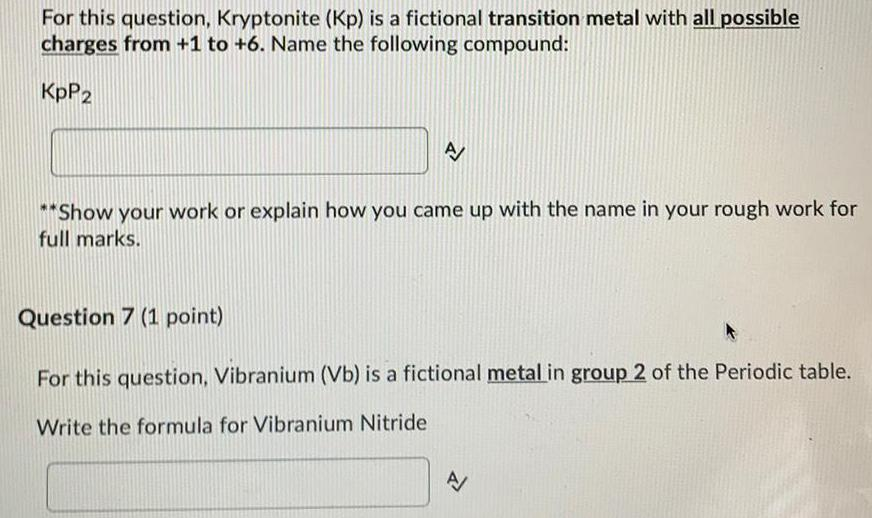Inorganic Chemistry
Metallurgy
For this question, Kryptonite (Kp) is a fictional transition metal with all possible charges from +1 to +6. Name the following compound: KpP2 For this question, Vibranium (Vb) is a fictional metal in group 2 of the Periodic table. Write the formula for Vibranium Nitride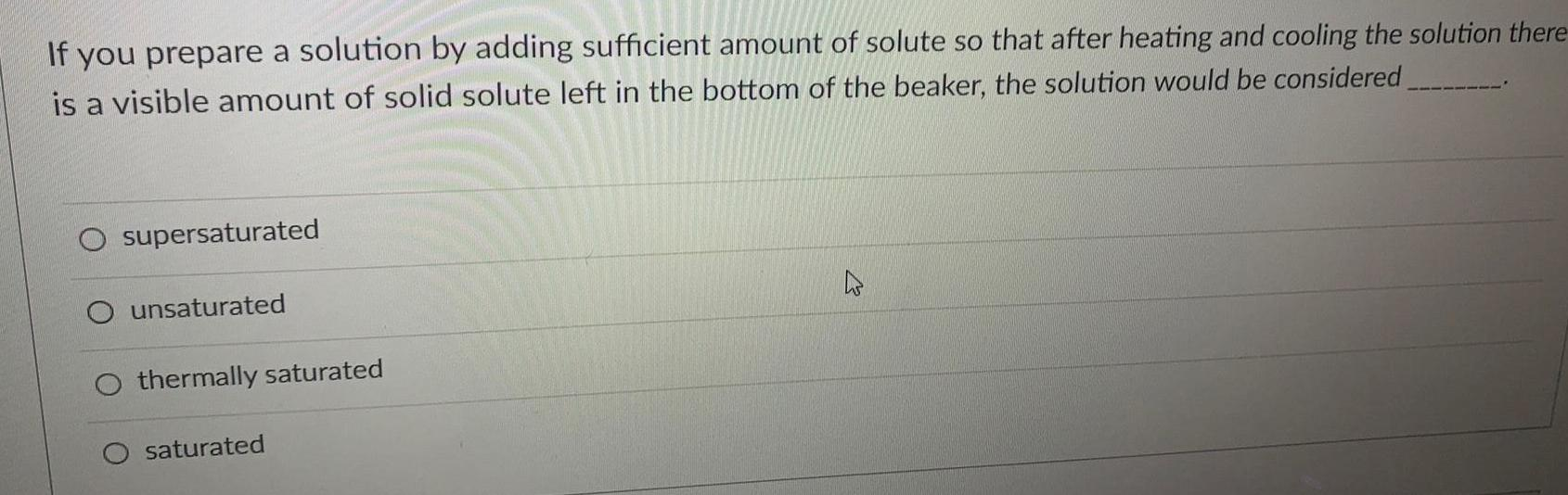Inorganic Chemistry
Metallurgy
If you prepare a solution by adding sufficient amount of solute so that after heating and cooling the solution there is a visible amount of solid solute left in the bottom of the beaker, the solution would be considered _______ supersaturated unsaturated thermally saturated saturated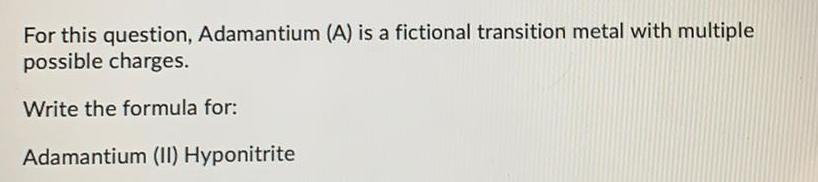Inorganic Chemistry
Metallurgy
For this question, Adamantium (A) is a fictional transition metal with multiple possible charges. Write the formula for: Adamantium (II) Hyponitrite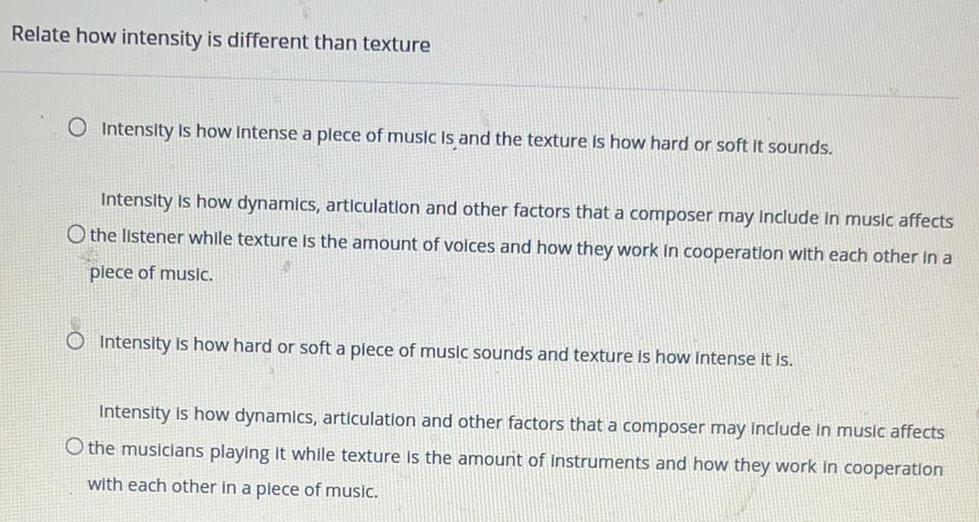Inorganic Chemistry
Metallurgy
Relate how intensity is different than texture Intensity is how Intense a plece of music Is and the texture is how hard or soft it sounds. Intensity is how dynamics, articulation and other factors that a composer may include in music affects the listener while texture is the amount of voices and how they work in cooperation with each other in a piece of music. Intensity is how hard or soft a piece of music sounds and texture is how intense it is. Intensity is how dynamics, articulation and other factors that a composer may include in music affects the musicians playing it while texture is the amount of instr and how they work in cooperation with each other in a plece of music.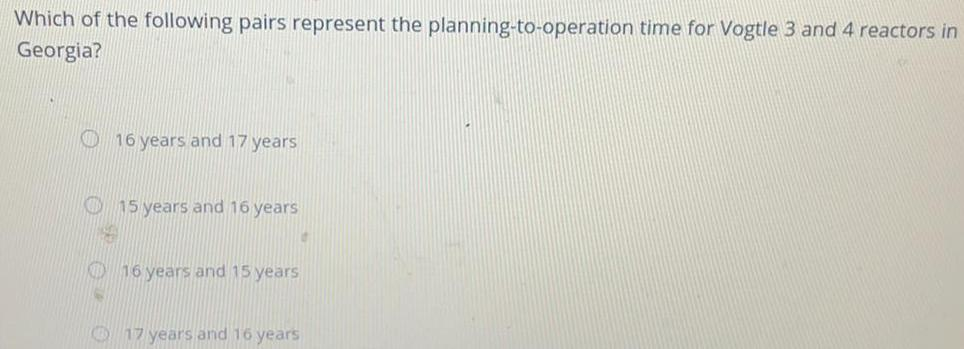Inorganic Chemistry
Metallurgy
Which of the following pairs represent the planning-to-operation time for Vogtle 3 and 4 reactors in Georgia? 16 years and 17 years 15 years and 16 years 16 years and 15 years 17 years and 16 years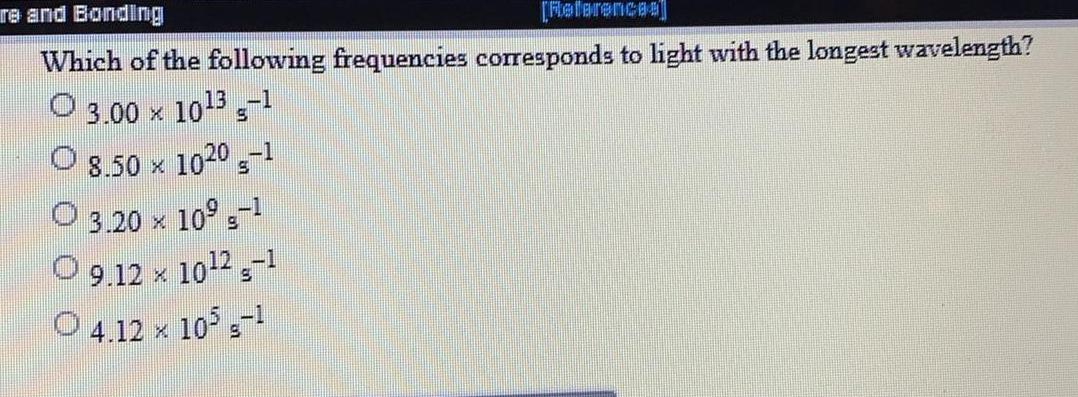Inorganic Chemistry
Metallurgy
Which of the following frequencies corresponds to light with the longest wavelength? 3.00 × 10^13 s^-1 8.50 x 10^20 s^-1 3.20 × 10^9 s^-1 9.12 x 10^12 s^-1 4.12 × 10^5 s^-1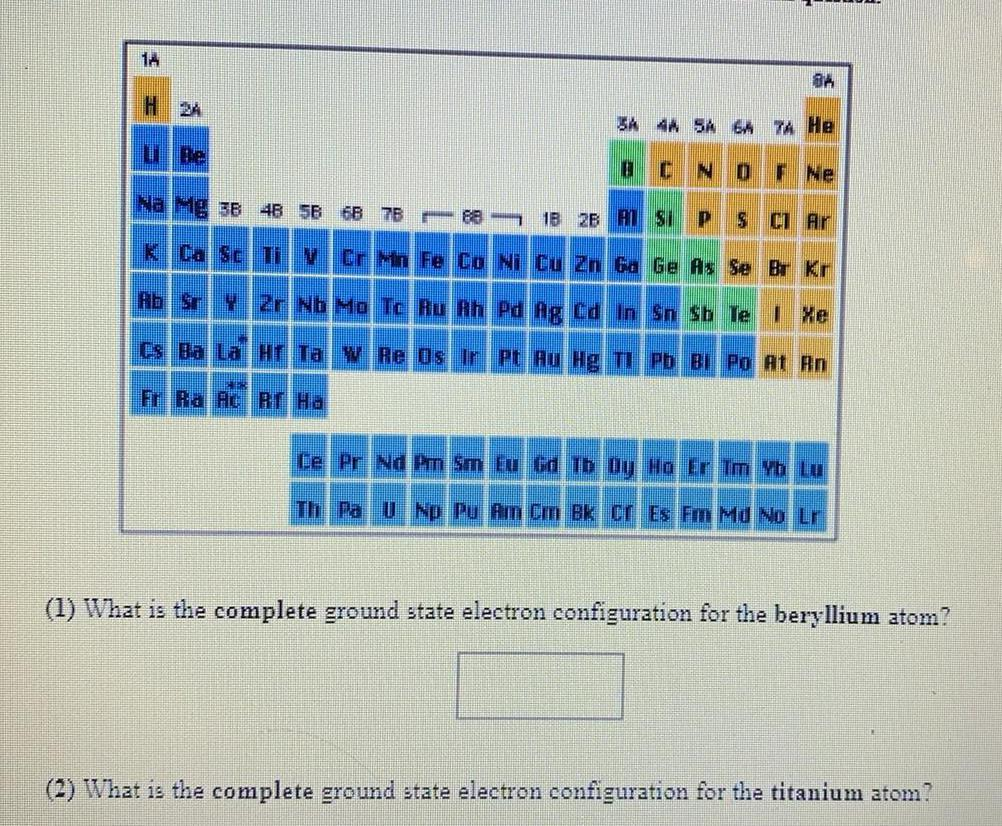Inorganic Chemistry
Metallurgy
1) What is the complete ground state electron configuration for the beryllium atom? (2) What is the complete ground state electron configuration for the titanium atom?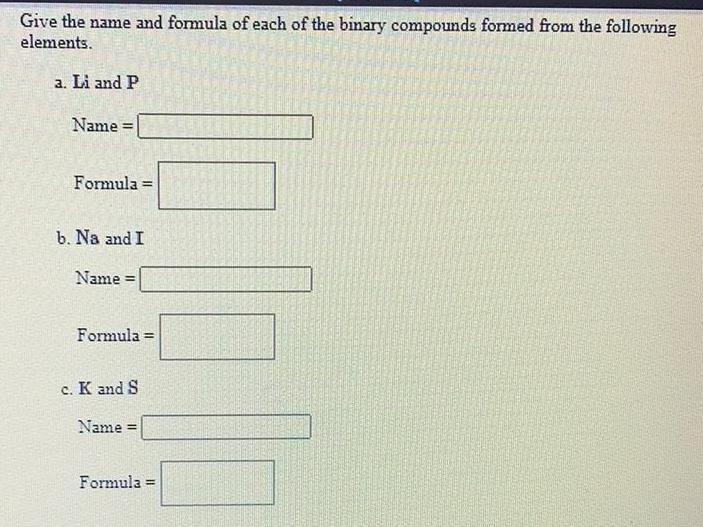Inorganic Chemistry
Metallurgy
Give the name and formula of each of the binary compounds formed from the following elements. a. Li and P Name = Formula = b. Na and I Name = Formula = c. K and S Name: = Formula =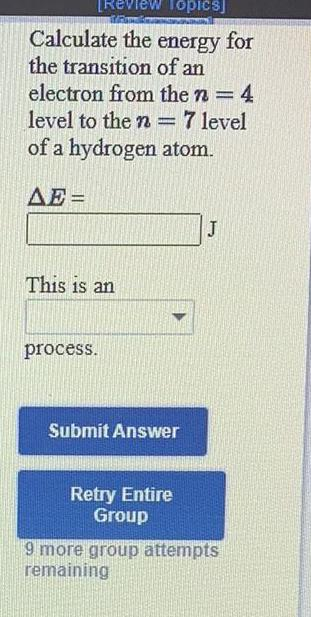Inorganic Chemistry
Metallurgy
Calculate the energy for the transition of an electron from the n = 4 level to the n = 7 level of a hydrogen atom.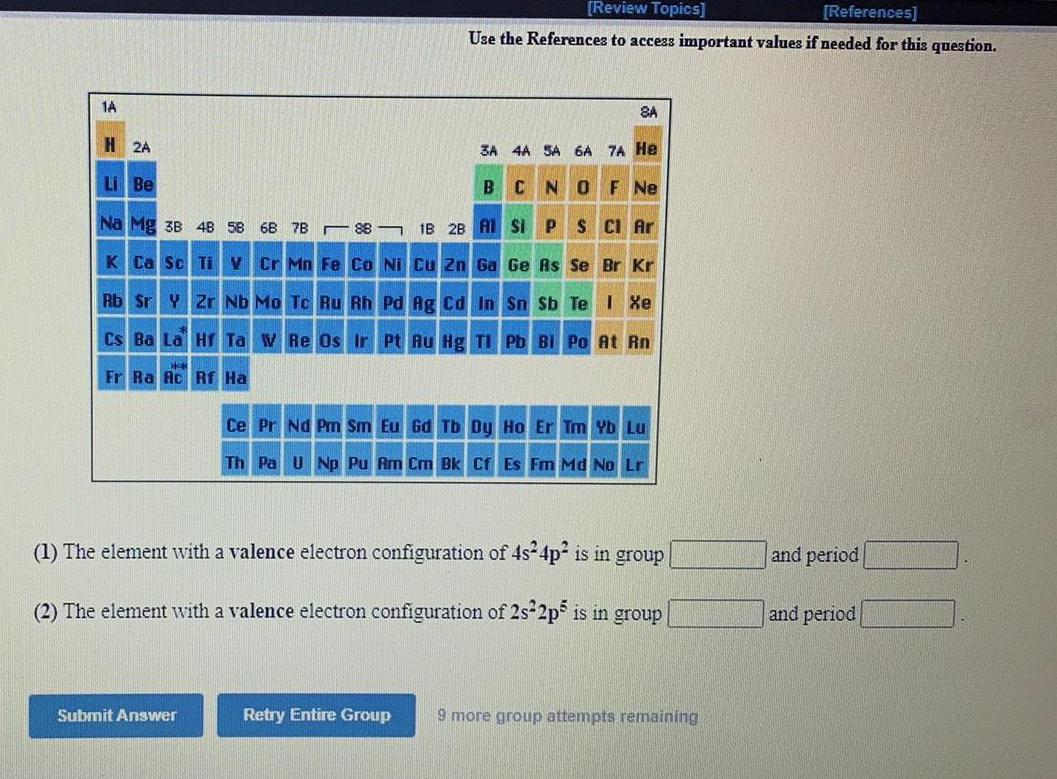Inorganic Chemistry
Metallurgy
(1) The element with a valence electron configuration of 4s²4p² is in group ____ and period ____ (2) The element with a valence electron configuration of 2s²2p5 is in group ____ and period ____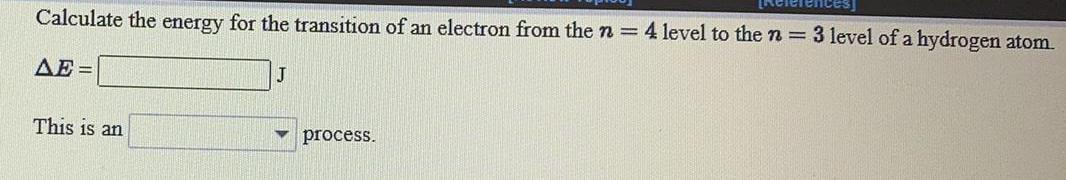Inorganic Chemistry
Metallurgy
Calculate the energy for the transition of an electron from the n=4 level to the n = 3 level of a hydrogen atom. ΔE= This is an _________ process.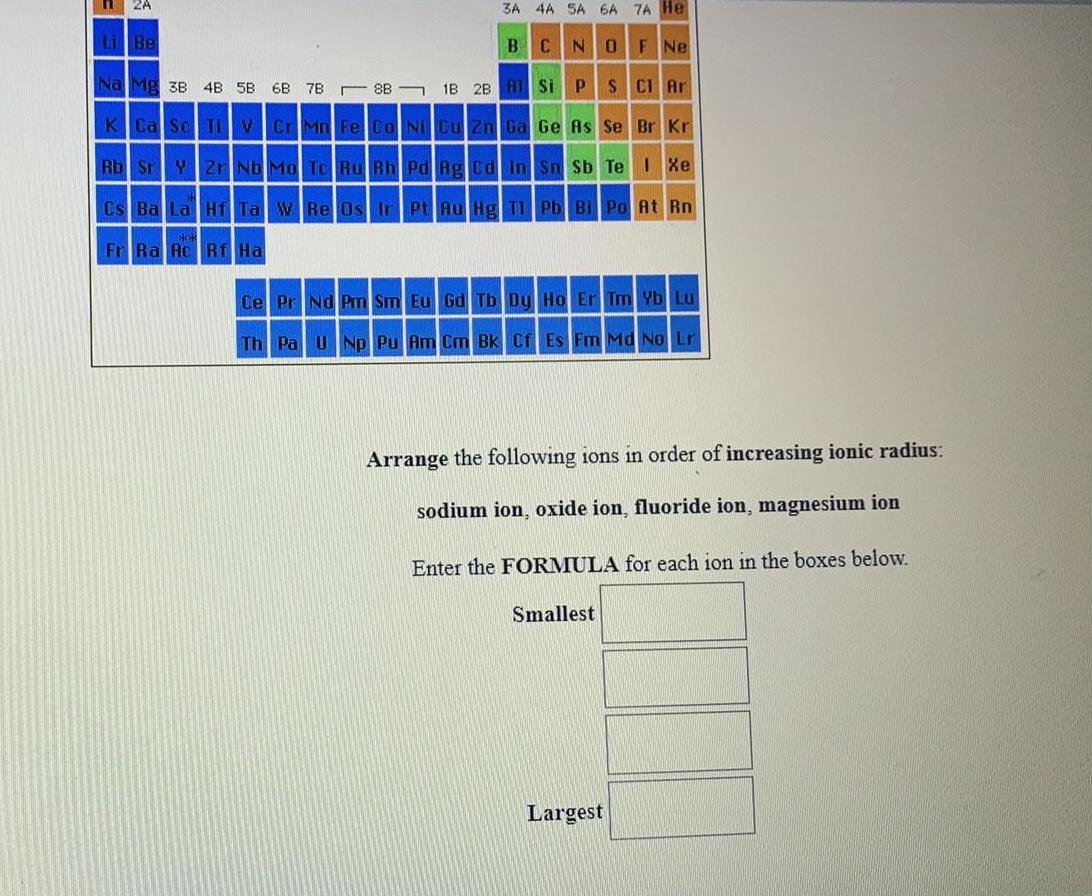Inorganic Chemistry
Metallurgy
Arrange the following ions in order of increasing ionic radius: sodium ion, oxide ion, fluoride ion, magnesium ion Enter the FORMULA for each ion in the boxes below. Smallest Largest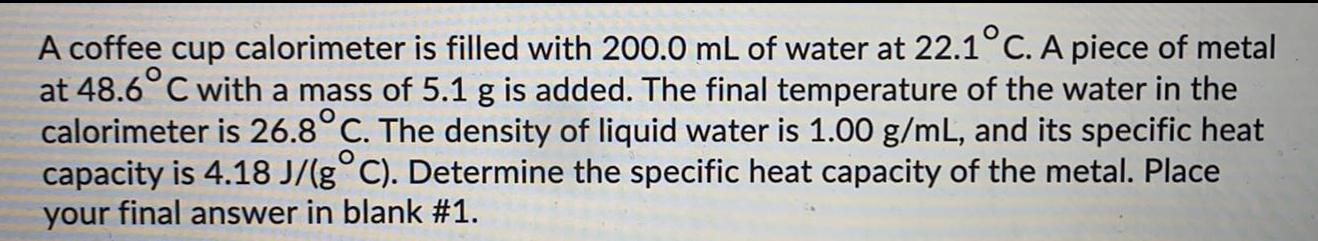Inorganic Chemistry
Metallurgy
A coffee cup calorimeter is filled with 200.0 mL of water at 22.1°C. A piece of metal at 48.6° C with a mass of 5.1 g is added. The final temperature of the water in the calorimeter is 26.8°C. The density of liquid water is 1.00 g/mL, and its specific heat capacity is 4.18 J/(g°C). Determine the specific heat capacity of the metal. Place your final answer in blank #1.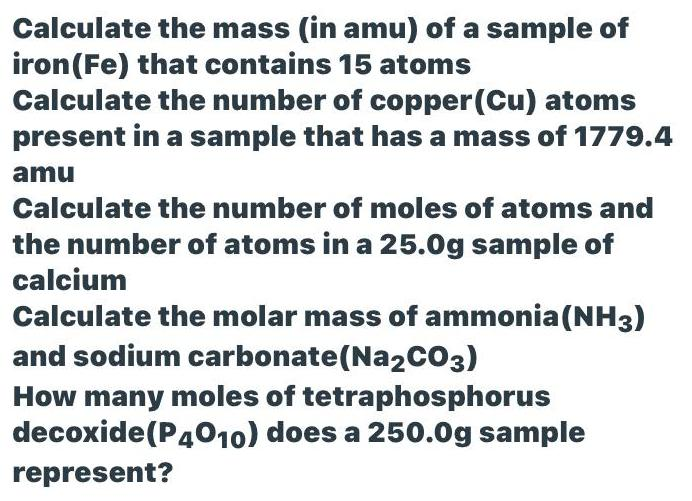Inorganic Chemistry
Metallurgy
Calculate the mass (in amu) of a sample of iron (Fe) that contains 15 atoms Calculate the number of copper (Cu) atoms present in a sample that has a mass of 1779.4 amu Calculate the number of moles of atoms and the number of atoms in a 25.0g sample of calcium Calculate the molar mass of ammonia (NH3) and sodium carbonate (Na2CO3) How many moles of tetraphosphorus decoxide (P4010) does a 250.0g sample represent?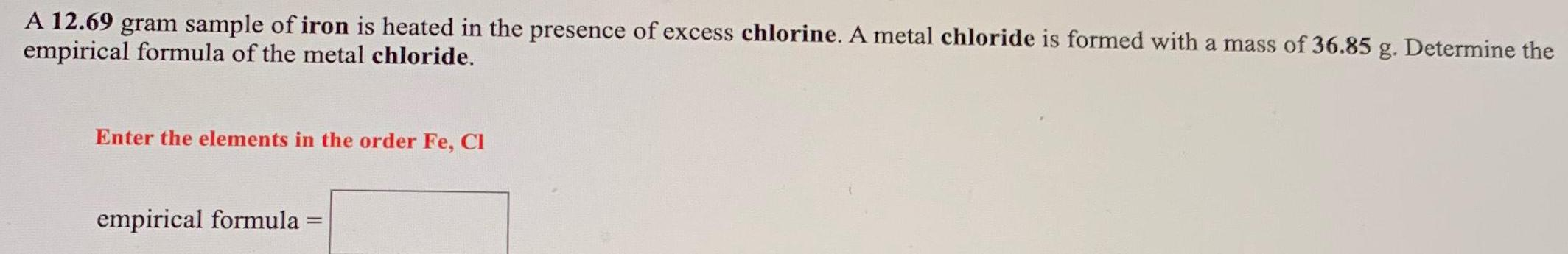Inorganic Chemistry
Metallurgy
A 12.69 gram sample of iron is heated in the presence of excess chlorine. A metal chloride is formed with a mass of 36.85 g. Determine the empirical formula of the metal chloride.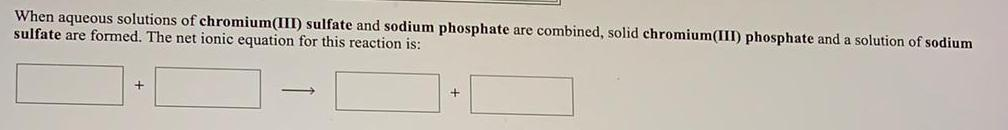Inorganic Chemistry
Metallurgy
When aqueous solutions of chromium(III) sulfate and sodium phosphate are combined, solid chromium(III) phosphate and a solution of sodium sulfate are formed. The net ionic equation for this reaction is: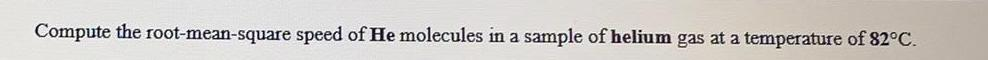Inorganic Chemistry
Metallurgy
Compute the root-mean-square speed of He molecules in a sample of helium gas at a temperature of 82°C.